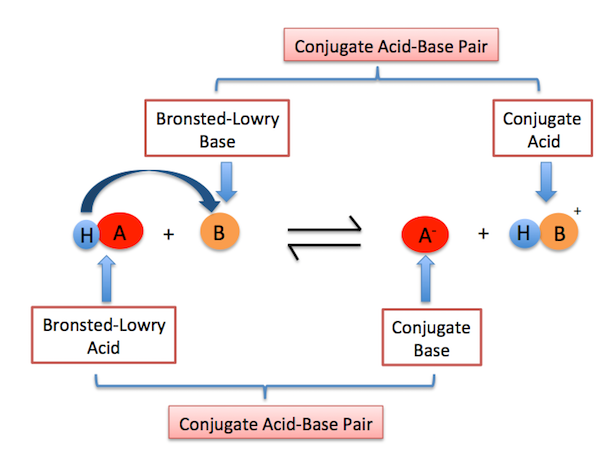# How are Bronsted lowry acid and bases defined?

Aug 21, 2016

A Brønsted-Lowry acid is any substance (molecule or ion) that can donate a hydrogen ion (${H}^{+}$).

A Brønsted-Lowry base is any species that can accept a hydrogen ion (${H}^{+}$).

#### Explanation:

In short, acids have the ability to donate protons and bases have the ability to accept protons.

Take the following reaction for example:

${H}_{2} S {O}_{4} + N {H}_{3} r i g h t \le f t h a r p \infty n s N {H}_{4}^{+} + H S {O}_{4}^{-}$

Sulfuric acid (${H}_{2} S {O}_{4}$) is the Brønsted-Lowry acid because it donates a hydrogen ion. Ammonia ($N {H}_{3}$) is the Brønsted-Lowry base because it accepts the hydrogen ion.

The Brønsted-Lowry theory also introduces the concept of conjugate acid-base pairs. A conjugate acid-base pair are two species that differ by a (${H}^{+}$) ion.

Based on the reaction above, the ammonium ion
($N {H}_{4}^{+}$) is the conjugate acid of the base ammonia and the hydrogen sulfate ion ($H S {O}_{4}^{-}$) is the conjugate base of sulfuric acid.

Here's a tip to let you know which substance is the conjugate acid and which substance is the conjugate base:

A conjugate base has one less (${H}^{+}$) proton than the acid you started with.

A conjugate acid has one more (${H}^{+}$) proton than the base you started with.

Here's general depiction of conjugate acid-base pairs:$H A$ represents an acid

$B$ represents a base

${A}^{-}$ represents the conjugate base since this substance has one less proton than the acid, HA.

$H {B}^{+}$ represents the conjugate acid since this substance has one more proton than the base, B.

I really hope this makes sense!